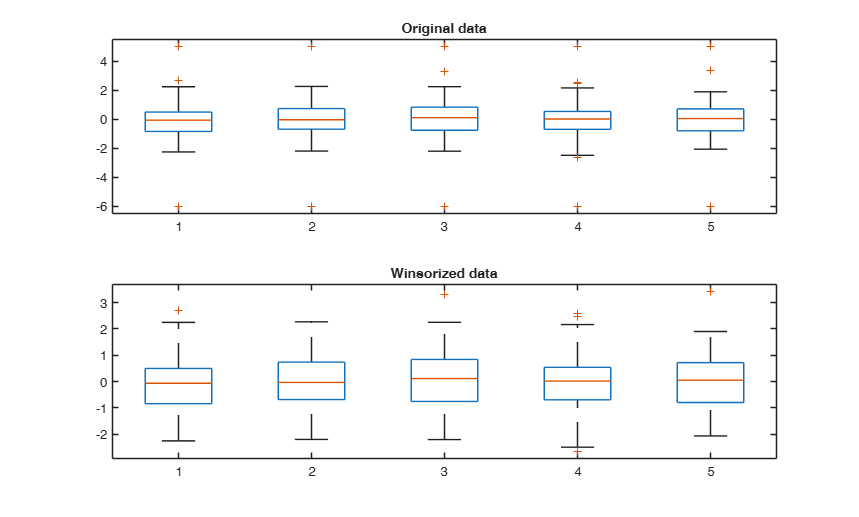winsor

winsor returns a winsorized copy of input

Description

Xwins =winsor(X, p) Winsorize each column of matrix X.

Xwins =winsor(X, p, dim) Winsorize each row of 3D array X.

Examples

expand allWinsorize each column of matrix X.

Reinitialize the random number generator to its startup configuration.

rng('default')
rng(100)
X = randn(100,5);
% Contaminate 5th and 10th row of matrix X
X(5,:)=5;
X(10,:)=-6;
p=[1 99];
Y = winsor(X,p);
subplot(2,1,1)
boxplot(X)
title('Original data')
subplot(2,1,2)
boxplot(Y)
title('Winsorized data')Winsorize each row of 3D array X.

rng(100)
X = randn(20,100,3);
p=[1 99];
Y = winsor(X,p,2);
disp('Number of unique elements in second rows of first slice of 3D array X')
disp(length(unique(Y(2,:,1)')))

Input Arguments

X — Input array. Vector | matrix | 3D array.

Input array, specified as a vector, matrix, or 3D array.

Data Types: ingle | double | int8 | int16 | int32 | int64 |uint8 | uint16 | uint32 | uint64

p — Percentiles to use to winsorize. Vector of length 2 with elements in the interval [0 100].

Vector of length(2) containing cut-off percentiles (left, right). Note that p(2) must be greater than p(1).

Data Types: single | double | int8 | int16 | int32 | int64 |uint8 | uint16 | uint32 | uint64

dim — Dimension to operate along. Positive integer scalar.

If no value is specified, then the default is the first array dimension whose size does not equal 1. In other words, if the input is a row (column) vector it winsorizes this row (column) vector. If the input is a nxp matrix it winsorizes the columns. If the input is a 3D arrary of size nxpxq it winsorizes each column of each slice of the 3D array.

Example: 2

Data Types: ingle | double | int8 | int16 | int32 | int64 |uint8 | uint16 | uint32 | uint64

Output Arguments

Xwins —X winsorized. Array of the same size of X

In array Xwins all entries smaller than the p(1) quantile have been replaced by this value and likewise for all entries larger than the p(2) quantile. Row, or columns are winsorized depending on optional input scalar dim.

Maronna, R.A., Martin D. and Yohai V.J. (2006), "Robust Statistics, Theory and Methods", Wiley, New York.